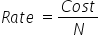Mathematics
Easy

Question

# Four gallons of gasoline costs \$16.80. The cost of 15 gallons would be ________.

## 60635053Hint:

## The correct answer is: 63

### Let’s denote number of gallons by N.The given values of number of gallons of gasoline and cost are as follows.N = 4Cost = \$16.80We have to find the cost of 15 gallons. We will find the rate first. We will find rate by taking the ratio of cost to the number of gallons. As rate is constant, we will use it to find the cost of 15 gallons.Let’s see the equationWe will use this value to find the cost of 15 gallonsLet’s see the equationWe will rearrange the expression for N. We will multiply L.H.S and R.H.S by N.Rate × N = CostCost = Rate × N= 4.2 × 15= 63 dollarsTherefore, the cost of 15 gallons is 63 dollars.

The other method is to take the ratios and directly equate them. As the rate is constant, so the ratios will be constant. So we will consider the quantity we have to find as x and then equate the ratios. It will be a shortcut.

### Related Questions to study#### With Turito Foundation.#### Get an Expert Advice From Turito.